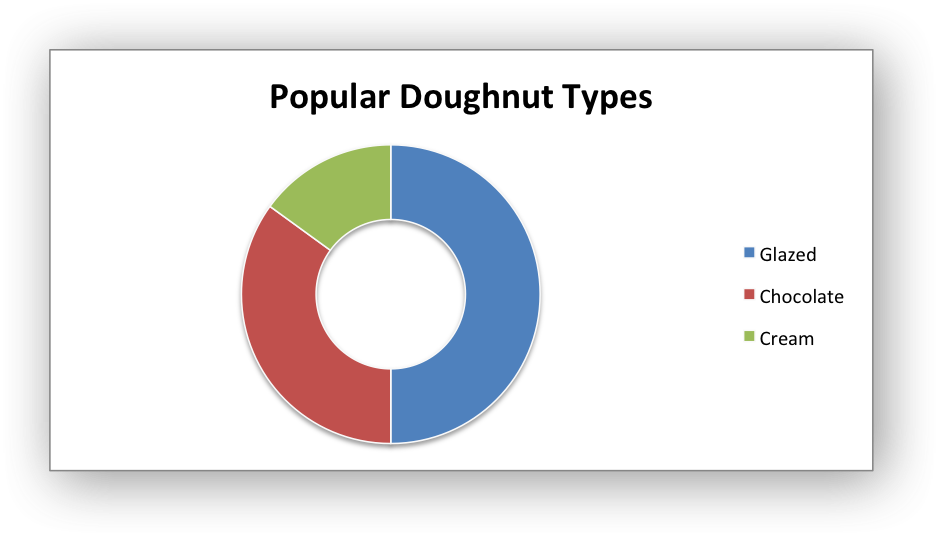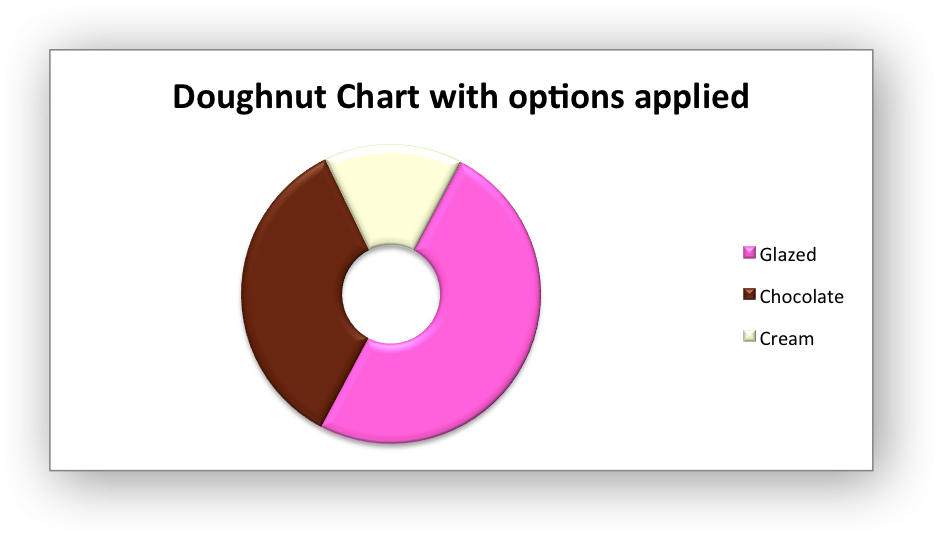# Example: Doughnut Chart

Example of creating Excel Doughnut charts. Chart 1 in the following example is:Chart 4 shows how to set segment colors and other options.

It is possible to define chart colors for most types of XlsxWriter charts via the `add_series()` method. However, Pie/Doughnut charts are a special case since each segment is represented as a point and as such it is necessary to assign formatting to each point in the series.```#######################################################################
#
# An example of creating Excel Doughnut charts with Python and XlsxWriter.
#
# The demo also shows how to set segment colors. It is possible to
# define chart colors for most types of XlsxWriter charts
# via the add_series() method. However, Pie/Doughnut charts are a special
# case since each segment is represented as a point so it is necessary to
# assign formatting to each point in the series.
#
# Copyright 2013-2022, John McNamara, jmcnamara@cpan.org
#
import xlsxwriter

workbook = xlsxwriter.Workbook('chart_doughnut.xlsx')

bold = workbook.add_format({'bold': 1})

# Add the worksheet data that the charts will refer to.
headings = ['Category', 'Values']
data = [
['Glazed', 'Chocolate', 'Cream'],
[50, 35, 15],
]

worksheet.write_column('A2', data)
worksheet.write_column('B2', data)

#######################################################################
#
# Create a new chart object.
#
chart1 = workbook.add_chart({'type': 'doughnut'})

# Configure the series. Note the use of the list syntax to define ranges:
'name':       'Doughnut sales data',
'categories': ['Sheet1', 1, 0, 3, 0],
'values':     ['Sheet1', 1, 1, 3, 1],
})

# Add a title.
chart1.set_title({'name': 'Popular Doughnut Types'})

# Set an Excel chart style. Colors with white outline and shadow.
chart1.set_style(10)

# Insert the chart into the worksheet (with an offset).
worksheet.insert_chart('C2', chart1, {'x_offset': 25, 'y_offset': 10})

#######################################################################
#
# Create a Doughnut chart with user defined segment colors.
#

# Create an example Doughnut chart like above.
chart2 = workbook.add_chart({'type': 'doughnut'})

# Configure the series and add user defined segment colors.
'name': 'Doughnut sales data',
'categories': '=Sheet1!\$A\$2:\$A\$4',
'values':     '=Sheet1!\$B\$2:\$B\$4',
'points': [
{'fill': {'color': '#FA58D0'}},
{'fill': {'color': '#61210B'}},
{'fill': {'color': '#F5F6CE'}},
],
})

# Add a title.
chart2.set_title({'name': 'Doughnut Chart with user defined colors'})

# Insert the chart into the worksheet (with an offset).
worksheet.insert_chart('C18', chart2, {'x_offset': 25, 'y_offset': 10})

#######################################################################
#
# Create a Doughnut chart with rotation of the segments.
#

# Create an example Doughnut chart like above.
chart3 = workbook.add_chart({'type': 'doughnut'})

# Configure the series.
'name': 'Doughnut sales data',
'categories': '=Sheet1!\$A\$2:\$A\$4',
'values':     '=Sheet1!\$B\$2:\$B\$4',
})

# Add a title.
chart3.set_title({'name': 'Doughnut Chart with segment rotation'})

# Change the angle/rotation of the first segment.
chart3.set_rotation(90)

# Insert the chart into the worksheet (with an offset).
worksheet.insert_chart('C34', chart3, {'x_offset': 25, 'y_offset': 10})

#######################################################################
#
# Create a Doughnut chart with user defined hole size and other options.
#

# Create an example Doughnut chart like above.
chart4 = workbook.add_chart({'type': 'doughnut'})

# Configure the series.
'name': 'Doughnut sales data',
'categories': '=Sheet1!\$A\$2:\$A\$4',
'values':     '=Sheet1!\$B\$2:\$B\$4',
'points': [
{'fill': {'color': '#FA58D0'}},
{'fill': {'color': '#61210B'}},
{'fill': {'color': '#F5F6CE'}},
],
})

# Set a 3D style.
chart4.set_style(26)

# Add a title.
chart4.set_title({'name': 'Doughnut Chart with options applied'})

# Change the angle/rotation of the first segment.
chart4.set_rotation(28)

# Change the hole size.
chart4.set_hole_size(33)

# Insert the chart into the worksheet (with an offset).
worksheet.insert_chart('C50', chart4, {'x_offset': 25, 'y_offset': 10})

workbook.close()
```